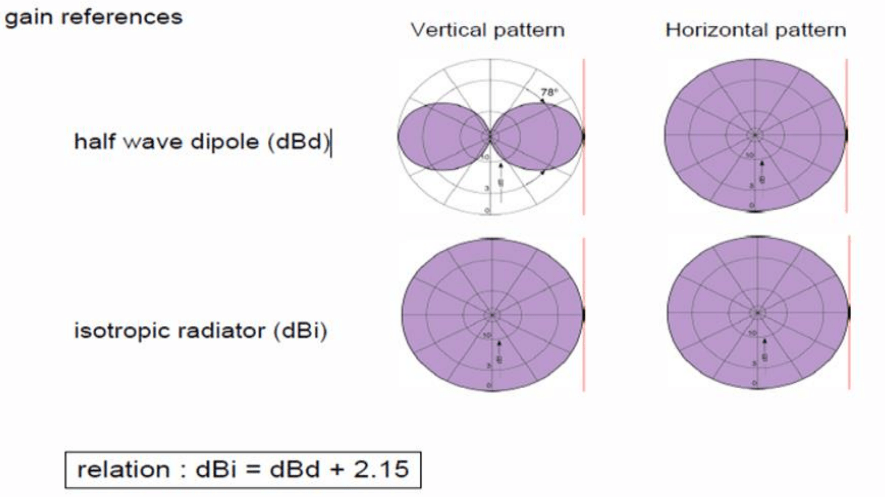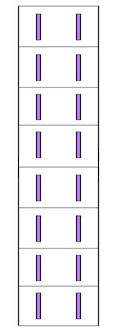# Antenna Gain and Antenna Beam Calculation

Antenna Gain is a parameter which measures the degree of directivity of the antenna’s radiation pattern. A high-gain antenna will preferentially radiate in a particular direction. The gain of an antenna is a passive phenomenon – power is not added by the antenna, but simply redistributed to provide more radiated power in a certain direction than would be transmitted by an isotropic antenna. The gain is measured in dBi and dBd.

• dBi is gain with reference to Isotropic Antenna
• dBd is gain with reference to Dipole Antenna

In practice, the half-wave dipole is taken as a reference instead of the isotropic radiator. The gain is then given in dBd (decibels over dipole):

There is a relation between dBd and dBi given below

• dBi= dBd + 2.15An antenna designer must take into account the application for the antenna when determining the gain.

• High-gain antennas have the advantage of longer range and better signal quality, but must be aimed carefully in a particular direction
• Low-gain antennas have shorter range, but the orientation of the antenna is relatively inconsequential

Antenna Gain and Dipole Arrangement
To concentrate the radiated power into the area around the horizon, half wave dipoles are arranged vertically and combined in phase With every doubling of the dipoles number.

• The half power beam width approx. halves
• The gain increases by 3 dB in the main direction

Following figure show the vertical arrangement of dipole beam width and respective gainAccordingly also in the horizontal plane a beam can be created with each halving of the beam width the gain is
increased by 3 dB (the shown patterns are theoretically).The resulting gain of an antenna is the sum of the “vertical” and “horizontal” gain

Calculation of the Gain and Beam width for an Antenna
An Antenna is in below figure in which dipoles are arranged in vertical polarization. Following are the step to calculate the gain of antenna. The shown Antenna has 8 Dipole in vertical direction which contributes 9dB. 3 dB due to Back plane of antenna and further 3 dB to one more column of 8 dipole in Horizontal plane.• 9 dB gain due to 8 no of dipoles in vertical direction
• 3 dB gain due to Backplane
• 3 dB gain due to addition of one more column of eight dipoles in horizontal plane

Total gain =9 +3 +3 = 15 dB with reference to dipole antenna

Now if we want to convert it with reference to the Isotropic antenna then we have to add 2.15 dB in gain of dipole
Then gain of antenna in dBi = 15 +2.15dB =17.15 dB

In the same manner we can find out the beam width of antenna.

A dipole have 78 Degree beam in vertical plane and doubling the dipole makes beam width half. For 2 Dipole in vertical make vertical beam width to 32 Degree and further 8 Dipole make beam width as 7 degree. Some of the beam power is wasted in the minor lobe while designing the antenna.

Same with Horizontal beam width, the two columns make horizontal beam width as 90 degree. Then the specification of such antenna becomes as below:

• Horizontal beam width = 90 Degree
• Vertical beam Width = 7 Degree
• Gain of antenna = 17.15 dBi